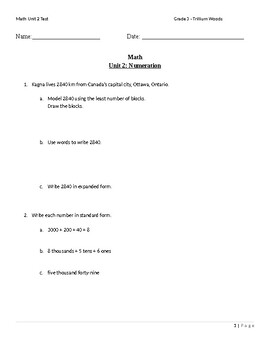# Numeration TestSubject
Resource Type
File Type

Word Document File

(17 KB)
Product Rating
Standards
• Product Description
• StandardsNEW

This test focuses on the Numeration Unit. Students will be required to model numbers up to thousands using base tens blocks, multiply by 10, 100,1000, and order numbers. Students will also be required to round to the nearest hundred. Students will also be asked to estimate dollar amount and calculate money in addition to knowing the different between standard and expanded form.

I have scored this test out of 18 with each question being worth 1 point

Use place value understanding to round multi-digit whole numbers to any place.
Read and write multi-digit whole numbers using base-ten numerals, number names, and expanded form. Compare two multi-digit numbers based on meanings of the digits in each place, using >, =, and < symbols to record the results of comparisons.
Recognize that in a multi-digit whole number, a digit in one place represents ten times what it represents in the place to its right. For example, recognize that 700 ÷ 70 = 10 by applying concepts of place value and division.
Multiply one-digit whole numbers by multiples of 10 in the range 10–90 (e.g., 9 × 80, 5 × 60) using strategies based on place value and properties of operations.
Fluently add and subtract within 1000 using strategies and algorithms based on place value, properties of operations, and/or the relationship between addition and subtraction.
Total Pages
N/A
N/A
Teaching Duration
N/A
Report this Resource to TpT
Reported resources will be reviewed by our team. Report this resource to let us know if this resource violates TpT’s content guidelines.